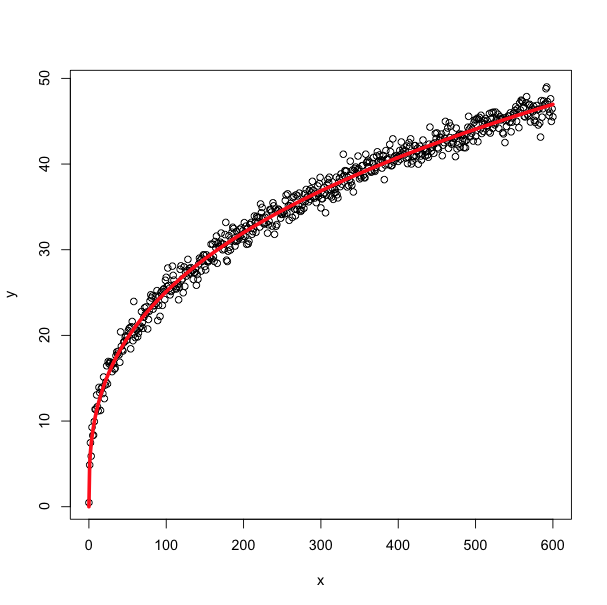Recent Tutorials and Articles
Getting Started with TensorFlow
Published on: 25th May 2018
Posted By: Amit Kumar

This tutorial introduces you to TensorFlow Deep learning framework including its installation, use cases and a hello world program.

# Introduction to TensorFlow

TensorFlow was developed in C and C++ language by Google Brain Team. It was primarily developed for running the operations involving heavy computations. It can execute computation heavy operations on variety of processor devices such as CPU, GPU, Cloud TPU etc using CUDA parallel computing platform APIs.

Since this library can deal with heavy computations tasks, this was deemed to be suited for machine learning applications. Infact, TensorFlow is now known as library for creating large scale machine or deep learning applications.

Although TensorFlow is written in C and C++, it provides APIs for both Python and C++. Infact, Python API is recommended as it is more complete and sophisticated than C++ one.

# Primitives of TensorFlow

TensorFlow let us define set of interrelated (or series of) operations using Data Flow Graphs data structure. Just like any graph, there are two parts of it -

1. Nodes - These represent mathematical operations like step function, logistic function or any other mathematical function
2. Edges - These represent multi-dimensional array of data called Tensors that is passed from one node (mathematical operation) to another one.

Here is a very simple example of Data flow graph -Data flow graphs are executed with the help of a session. Session translates the graph and passes those to underlying processing device for execution.

# Installing TensorFlow

Since Python API is recommended one, we will see how to go about TensorFlow in Python. Installing TensorFlow in Python is as simple as running following command -

``pip install tensorflow``

This will also install Tensorboard application that can be used to visualize and debug data flow graphs.

# Hello World Program in TensorFlow

Once we have installed TensorFlow, let's start with writing a simple program of multiplying two matrices as below -

``````# import tensorflow library with alias tf
import tensorflow as tf

# Define two random matrices of 2X2 dimension
matrix1 = tf.constant([[1, 2], [4, 5]], name="Matrix1")
matrix2 = tf.constant([[7, 8], [1, 2]], name="Matrix2")

# Multiply two matrices
result = tf.matmul(matrix1, matrix2)

# Get session and execute above operation in Session
session = tf.Session()
print session.run(result)

# Serialize graph to current directory to visualize into Tensorboard
writer = tf.summary.FileWriter('.')
``````

Output:

``````[[ 9 12]
[33 42]]
``````

Since we have serialized our graph data using TensorFlow summary filewriter, we can also visualize data flow graph of above diagram using following command from same directory the above program was executed -

``````# logdir is specified as current directory as this is where we serialized graph in above example
tensorboard --logdir .``````

With above command, Tensorboard application will be started and you will be able to access the graph at http://localhost:6006.

# Use Cases of TensorFlow

While TensorFlow can be used for many types of applications, here are some of famous use cases of TensorFlow -

• Linear Regression - TensorFlow can be used to create linear models to fit the training data samples into a line.• Non-linear Regression - In some scenario, linear models may not provide optimized fitment of data and require non-linear regression models. TensorFlow can also be used to create non-linear models.• Logistic Regression - TensorFlow also finds its application in classification problems by utilizing logistic function for Logistic Regression.
• Neural Networks - One of the famous use case of TensorFlow is building of neural network models as it provides faster execution time and necessary tools.
• And many more...

Thank you for reading through the tutorial. In case of any feedback/questions/concerns, you can communicate same to us through your comments and we shall get back to you as soon as possible.

Posted By: Amit Kumar
Published on: 25th May 2018